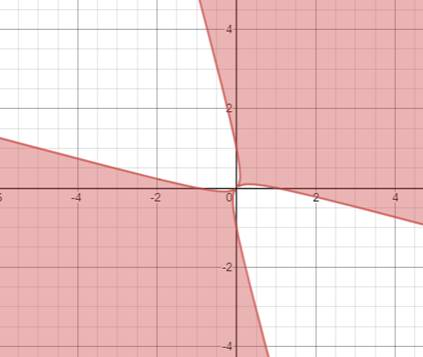# The region in the plane consisting of all points x , y### Single Variable Calculus: Concepts...

4th Edition
James Stewart
Publisher: Cengage Learning
ISBN: 9781337687805### Single Variable Calculus: Concepts...

4th Edition
James Stewart
Publisher: Cengage Learning
ISBN: 9781337687805

#### Solutions

Chapter 4, Problem 10P
To determine

## The region in the plane consisting of all points x,y

Expert Solution

### Explanation of Solution

Given information:

2xy|xy|x2+y2 .

Calculations:

Now here it is given,

2xy|xy|x2+y2

Therefore it can be written as,

2xyx2+y2x2+y22xyx2+y22xy0 (xy) 2 0

Therefore the interval can be written as,

0|xy|2xy(xy)2

Using the values of the interval and using a graphing tool we draw the graph below.

The shaded region shows the values above zero in the interval.### Have a homework question?

Subscribe to bartleby learn! Ask subject matter experts 30 homework questions each month. Plus, you’ll have access to millions of step-by-step textbook answers!